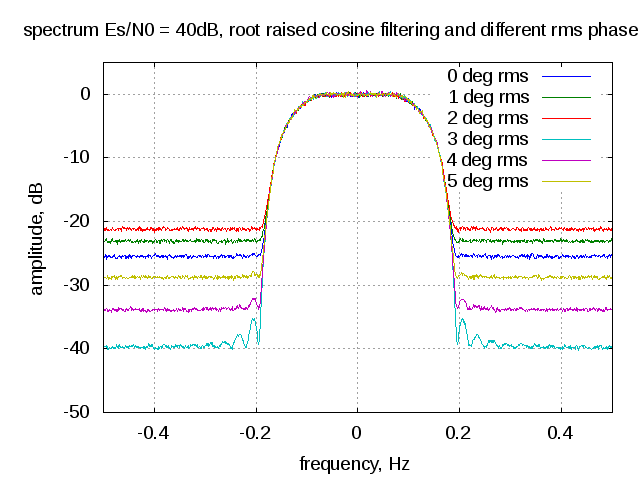- DSP log - http://www.dsplog.com -

Transmit spectrum with phase noise

Posted By Krishna Sankar On August 28, 2012 @ 6:07 am In Analog | No Comments

The earlier posts on phase noise discussed about phase noise in oscillators , conversion of phase noise profile to jitter  and the impact of phase noise on the error vector magnitude (evm) . This post discuss the impact of phase noise on the spectrum of the transmit waveform.  A simple random QPSK modulated symbols, oversampled and passed through a root raised cosine filtering is used for the simulation.

System Model

Consider a simple system model having both phase noise and thermal noise as show below.Figure: System model with phase and thermal noise

The received symbol is, , where is the phase distortion in radians,  is the transmit symbol and  is the contribution due to thermal noise The phase is Gaussian distributed with zero mean and variance  radians^2 having a probability density function as, . From the post on phase noise on the error vector magnitude (evm) , we know that the

Es/N0 = 40dB

 Phase Noise (deg, rms) EVM, dB 0 -40.00 1 -33.93 2 -28.80 3 -25.46 4 -23.03 5 -21.13

Table : Resultant EVM with phase noise and thermal noise

Further, from the post on phase noise in oscillators , it is also known that the phase noise will cause skirts in the spectrum of the carrier.Figure : Spectrum on the transmit waveform with random QPSK symbols, root raised cosine filtering (oversampling by 4), Es/N0 = 40dB and different RMS phase noise. ObservationsAs can be seen from the above plot, the phase noise elevates the out-of band emissions in the transmit spectrum (from -40dB in the no phase noise case to -21.13dB for the 5 degree rms phase) So it is important to have a lower phase noise to meet the spectral mask specification in addition to minimize the impact on error vector magnitude.

Matlab/Octave code

Attached script computes the transmit spectrum of a QPSK modulated symbol versus Es/N0 for different values of rms phase noise.

% Script for simulating the transmit spectrum of a QPSK
% modulated symbol affected by phase noise and thermal noise
% ----------------------------------------------------------

clear;close all;
N = 10^5; % number of symbols
os = 4; % oversampling factor

Es_N0_dB = 40;
phi_rms_deg_vec = [0:1:5];

% root raised cosine filter
t_by_Ts = [-4:1/os:4];
beta = 0.5;
ht = (sin(pi*t_by_Ts*(1-beta)) + 4*beta*t_by_Ts.*cos(pi*t_by_Ts*(1+beta)))./(pi*t_by_Ts.*(1-(4*beta*t_by_Ts).^2));
ht((length(t_by_Ts)-1)/2+1) = 1 -beta + 4*beta/pi;
ht([-os/(4*beta) os/(4*beta)]+(length(t_by_Ts)-1)/2+1) = beta/sqrt(2)*((1+2/pi)*sin(pi/(4*beta))+(1-2/pi)*cos(pi/(4*beta)));
ht = ht/sqrt(os);

for ii = 1:length(Es_N0_dB)
for jj = 1:length(phi_rms_deg_vec)

% Transmitter
ip_re = rand(1,N)>0.5; % generating 0,1 with equal probability
ip_im = rand(1,N)>0.5; % generating 0,1 with equal probability
s = 1/sqrt(2)*(2*ip_re-1 + j*(2*ip_im-1)); % QPSK modulation

% Pulse shaping
s_os = [s ; zeros(os-1,length(s))];
s_os = s_os(:).';
s_os = conv(ht,s_os);
s_os = s_os(1:os*N);

% Thermal and Phase Noise addition
n = 1/sqrt(2)*[randn(1,N*os) + j*randn(1,N*os)]; % thermal noise
phi = phi_rms_deg_vec(jj)*(pi/180)*randn(1,N*os)*sqrt(os); % phase noise
y = s_os.*exp(j*phi) + 10^(-Es_N0_dB(ii)/20)*n;

% computing the transmit spectrum
[Pxx1(jj,:) W2 ] = pwelch(y,[],[],1024,'twosided');

% matched filtering
y_mf_out = conv(y,fliplr(ht));
y_mf_out = y_mf_out(length(ht):os:end);

% error vector
error_vec = (y_mf_out-s);
evm(ii,jj)  = error_vec*error_vec';
theory_evm(ii,jj) =  10^(-Es_N0_dB(ii)/10) + 2 - 2*exp(-(phi_rms_deg_vec(jj)*pi/180).^2/2);

end
end

figure;
plot([-512:511]/1024,10*log10(fftshift(Pxx1)));
xlabel('frequency, Hz'); ylabel('amplitude, dB');
legend('0 deg rms','1 deg rms', '2 deg rms', '3 deg rms', '4 deg rms', '5 deg rms');
title('spectrum Es/N0 = 40dB, root raised cosine filtering and different rms phase noise');
axis([-0.5 0.5 -50 5]); grid on;

URL to article: http://www.dsplog.com/2012/08/28/transmit-spectrum-phase-noise/

URLs in this post:

 phase noise in oscillators: http://www.dsplog.com/2012/06/08/oscillator-phase-noise/

 conversion of phase noise profile to jitter: http://www.dsplog.com/2012/06/22/phase-noise-psd-to-jitter/

 phase noise on the error vector magnitude (evm): http://www.dsplog.com/2012/07/09/evm-phase-noise/